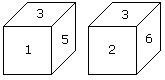# Verbal Reasoning - Dice - Discussion

### Discussion :: Dice - Dice 1 (Q.No.4)

4.

Two positions of a dice are shown below. Which number will appear on the face opposite to the face with the number 5?[A]. 2/6 [B]. 2 [C]. 6 [D]. 4

Explanation:

According to the rule no. (3), common faces with number 3, are in same positions. Hence the number of the opposite face to face with number 5 will be 6.

 Roxy said: (Jul 6, 2010) where is rule no.3

 Sundar said: (Jul 6, 2010) Hi Roxy, You can find it at http://www.indiabix.com/verbal-reasoning/dice/introduction Have a nice day!

 Jijo said: (Dec 4, 2010) The answer is option A) 2/6

 Narayana Rao Gartam said: (Jun 11, 2014) In two different positions of dice, 3 at same position and the dice has rotated in clockwise direction, all visible numbers are adjacent to the 3, the invisible number will be the opposite to number 5. Simple, if dice is rotates clockwise or anticlockwise without changing the top position. (Here is --- 3).

 Sufiyan said: (Aug 24, 2016) Option A is the right answer.

 Kamal Kishore B said: (Sep 21, 2016) Option C is the wrong answer, how it possible 6, can you explain?

 Shanthi said: (Nov 9, 2016) Please, explain clearly I can't understand why it's C not A.

 Sumit said: (Jan 5, 2017) The answer should be B 2 because this dice is standard dice and the sum of opposite face should be 7 in standard dice so 7-5 = 2. Tell me, where I am wrong.

 Anil said: (Jan 14, 2017) I think the question is framed wrongly as it is a standard die 5 have to be opposite 2 not 6.

 Venkatesh said: (Jan 16, 2017) Answer is B why beacuse it is general dice. General dice rule is each opposite addition becames 7. So, 1+6 = 7, 2+5 = 7, 3+4 = 7 so Ans is 2(b).

 Ritam said: (Feb 19, 2017) Option is D is right. How it is option C?

 Rashmi Chand, said: (Apr 13, 2017) It is standard dice. So the answer will be 2.

 Nilesh Guthriya said: (Jun 28, 2017) B is right.

 Priya Ranjan said: (Jul 7, 2017) The answer should be B) 2 because this dice is standard dice and the sum of opposite face should be 7.

 Ashish Patel said: (Aug 10, 2017) The correct Answer is 2.

 Deepak Maharana said: (Aug 17, 2017) Option (B) is right answer because it is a standard dice, hence 7-5=2 is the right answer.

 Mukul Bhardwaj said: (Aug 28, 2017) Answer c is correct if one no is same here 3 than fix 3 as a point and move clock wise and write all no and same format for 2nd dice 3 5 1. 3 6 2 here opposite of 5 is 6 and also rest no 4 is opposite of 3.

 Harish said: (Sep 12, 2017) Answer is 2. Beacuse it is a standard dice.

 Gulshan Singh said: (Oct 4, 2017) It's standard dice so answer is 2.

 Somesh said: (Nov 24, 2017) Correct answer is 2 because given dice is standard not an ordinary dice.

 Pramathesh said: (Jan 7, 2018) The answer should be option 'b'. As the sum of adjacent sides are not equal to 7 so the dice is of standard type. And in the case of standard dice the sum of two face opp. Sides are equal to 7 so answer should be 2. As, 5+2=7. Is this right or wrong?

 Rajbanshi said: (Feb 24, 2018) Right answer is 2. That is standard dice. So 5+2=7.

 Mubashar said: (Mar 5, 2018) Standard Dice so answer must be 2.

 Anomis said: (Apr 25, 2018) Option B is right answer because both are standard dice. So the sum of opposite Faces is 7. Hence opp face of 5 is 2.

 Arijit Dawnn said: (Jun 25, 2018) It is a Standard Dice (which means the sum of any two opposite sides is equal to 7). So, the answer will be 2. Thank you.

 Vikas said: (Jul 31, 2018) Yeah. It is a standard dice. So the answer must be 2.

 Sandip Pandit said: (Aug 11, 2018) The correct Answer will be 6. I agree with the given answer.

 Pavan Kalyaan Vysyaraju said: (Sep 18, 2018) Option A) 2/6 is the right answer. Because if the dice is standard then answer is 2 otherwise the answer will be 6.

 Prashann Chadar said: (Oct 4, 2018) Option B is the right answer. Because this is standard dice. So; 1-6. 2-5. 3-4.

 Sanjay said: (Oct 27, 2018) Option C is the right answer. Because general or standard dice both follow the rule no 3. It is not a standard dice.